Learn about Functions In Math – Definition, Types & more!

## Top Questions

t schedule 3 people for the day my % scheduled is 78.57% My question is how did the computer come up with the 78.57%?
View More

I would love to get a written solution. I did the other proof tree that you can see in the attaached files. So its supposed to look like that Thank You.
View More

xtbook. i really need in in half an hour
View More

## Let’s first understand what is a function?

A function refers to a set of rules, that provide a set of output values for each and every input value from its  domain. It relates each element of its domain (input) to exactly one element to its range (output).
A function is mostly represented asit is pronounced as, f of x.

## Example & Types of Functions:

A function could be broadly classified as Injective Function, Surjective Function, and Bijective Function.

### Injective Function:

It is also called a One-to-One function. It will have a unique output for each input. This does not mean that each output will have some input.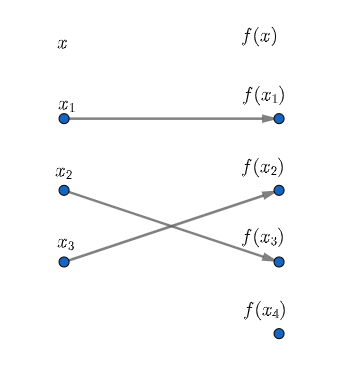### Surjective Function:

It is also called a Onto Function. It will have at least one input for every output. This means, for the same output, it could have more than one input.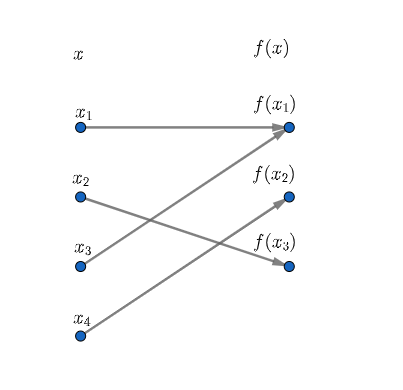### Bijective Function:

This is also called a One-to-One and Onto function. It will have exactly one output for each and every input.## Sample Questions of Function in Math:

Question 1: Analyze the following graph, and name the type of function.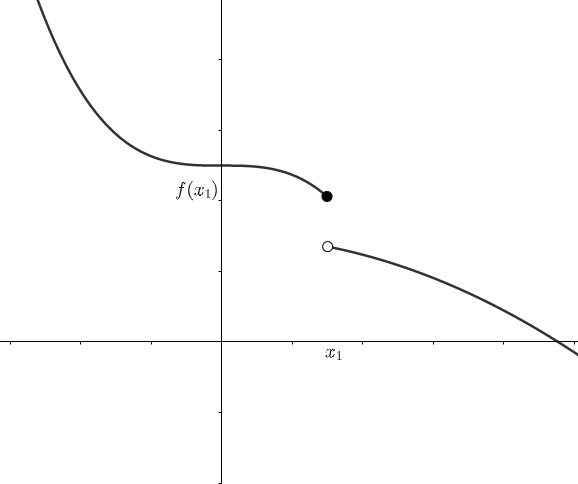Explanation: The given function is discontinuous at, before, and after the point, it has distinct input for distinct output.

However, it has an extra output represented by a white circle. This means it is an Injective Function.

Question 2: Analyze the following graph, and name the type of function.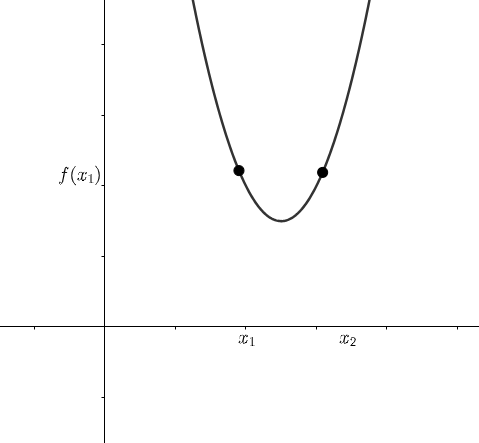Explanation: The given function has two inputsfor one output. This means it has more than one input for the same output. So, this is a surjective function.

Question 3: Analyze the following graph, and name the type of function.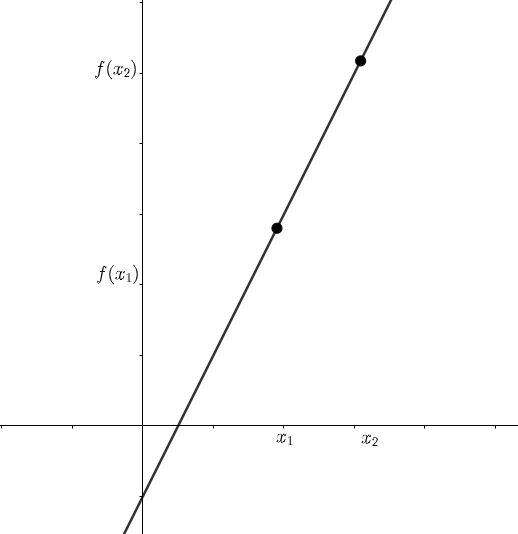Explanation: The following function has exactly one output for every input, so it will be a Bijective Function.

## Instant Functions Homework Help

Function is a high school math topic that is essential to learn and understand for every student. Wondering Why? Because Function in math is the only way by which we can calculate output based on the relation between various input variables. SAT math syllabus also includes questions on linear functions, quadratic functions, and Algebraic functions.

Functions can be considered as foundational blocks that help us do a lot of day to day operations from helping airplanes fly safely to creation of new machines and much more! Functions are also used by research for predicting natural disasters & by medical scientists for curing diseases.

For high school students, Functions Homework can be very challenging if the concept, definition, and basics are not strong. At school, many times students feel shy and don’t ask their teachers for more examples or details to understand the fundamentals clearly and start to fear the topic or subject completely.

We at TutorEye offer fast and reliable 24/7 homework help service for high school and college students and can take care of any difficult functions questions. Our virtual tutors are experienced and highly trained to detailed step-by-step guidance to homework questions.

We focus more on the process than on the end result to ensure that full transparency and clarity is provided to students on their homework assignments.

## Here are few simple steps to hire Math Homework Solver

Hire a math expert at reasonable price by following these few simple steps:

Step 1: Fill out the help homework form & upload a copy of your homework assignment.

Step 2: Align your budget and timelines by working with a tutor of your choice.

Question1: How to graph piecewise functions?

A piecewise function is one in which the output is defined by more than one formula. Every formula has its own domain, and the function's domain is the sum of these smaller domains.

To plot the graph of piecewise function

• Determine the boundaries on the x-axis given by each piece of the domain interval.
• Plot the graph with the help of the corresponding equation in its corresponding domain interval.
• Use dark circle point for closed interval and circle point for open interval.
• No two functions overlap each other.

Question2: How to graph rational functions?

For plotting graph of rational functions following steps should be followed:

• Find the x-intercept by equating numerator equal to zero and y-intercept by (0, f(0)).
• Set the denominator to zero and solve to find the vertical asymptotes.
• Using the fact above, find the horizontal asymptote if one exists.
• The number line will be divided into regions by the vertical asymptotes. At least one point in each area should be graphed.
• This point will tell us whether the graph will be above or below the horizontal asymptote, and we should obtain multiple points if necessary to ascertain the graph's overall form.
• Finally, plot the graph.

Question3: How to graph quadratic functions?

The quadratic equation is the function of degree 2. The graph of quadratic function is a parabola.

To plot the graph of quadratic functions following steps should be followed:

• Find the axis of symmetry
• Find the value of x and y intercept.
• Mark the (x,y) coordinate on the graph.

And finally, plot the graph.

Question4: How to solve functions?

For solving any function, we must find out the value of x. Now it depends on the type of the equation.

Let's take an example of linear function f(x) = ax+b

• In the problem, replace the value of f(x) with the value of f(x).
• To isolate the variable term, shift the constant term across the equal sign using the opposite sign procedure.
• Simplify and solve for x.

Question5: How to graph log functions?

For log function, characteristics of log must be followed:

1. One-to-one function
2. Vertical asymptote, i.e., x=0.
3. Range (−∞, ∞)
4. Domain (0, ∞)
5. X-intercept, i.e., (b,1)
6. Increasing function if b>1
7. Decreasing function 0 < b < 1

To plot the graph

• Draw the vertical asymptote, x = 0 and label it
• Plot the x-intercept, (1,0).
• Plot the key point (b,1).
• Connect the points with a smooth curve.

Finally, state the domain, (0,∞), the range, (−∞,∞), and the vertical asymptote, x =0.